Processing ......FreeComputerBooks.com Links to Free Computer, Mathematics, Technical Books all over the World

Beginning Mathematical Logic: A Study Guide
🌠 Top Free Python Books - 100% Free or Open Source!
• Title Beginning Mathematical Logic: A Study Guide
• Author(s) Peter Smith
• Publisher: Logic Matters (January 31, 2022)
• Paperback: 194 pages
• eBook: PDF
• Language: English
• ISBN-10/ASIN: 1916906338
• ISBN-13: 978-1916906334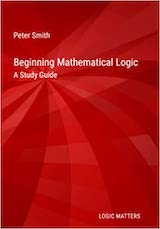Book Description

There are many wonderful introductory texts on mathematical logic, but there are also many not-so-useful books. So how do you find your way around the very large literature old and new, and how do you choose what to read? Beginning Mathematical Logic provides the necessary guide. It introduces the core topics and recommends the best books for studying these topics enjoyably and effectively. This will be an invaluable resource both for those wanting to teach themselves new areas of logic and for those looking for supplementary reading before or during a university course.

• Until he retired, Peter Smith taught logic at the University of Cambridge. His Logic Matters website and blog is at https://www.logicmatters.net.
Reviews, Ratings, and Recommendations: Related Book Categories: Read and Download Links:Similar Books:
•A Friendly Introduction to Mathematical Logic (Chris Leary)

In this user-friendly book, readers with no previous study in the field are introduced to the basics of model theory, proof theory, and computability theory, leading to rigorous proofs of Gödel's First and Second Incompleteness Theorems.

•Introduction to Mathematical Logic (Vilnis Detlovs, et al)

This book explores the principal topics of mathematical logic. It covers propositional logic, first-order logic, first-order number theory, axiomatic set theory, and the theory of computability. Discusses the major results of Gödel, Church, Kleene, Rosser, and Turing.

•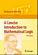A Concise Introduction to Mathematical Logic (W. Rautenberg)

This is a well-written introduction to the beautiful and coherent subject of mathematical logic. It contains classical material such as logical calculi, beginnings of model theory, and Goedel's incompleteness theorems, as well as some topics motivated by applications.

•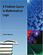A Problem Course in Mathematical Logic (Stefan Bilaniuk)

It is intended to serve as the text for an introduction to mathematical logic for undergraduates with some mathematical sophistication. It supplies definitions, statements of results, and problems, along with some explanations, examples, and hints.

•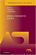Model-Theoretic Logics (Jon Barwise, et al)

This book brings together several directions of work in model theory between the late 1950s and early 1980s. It provides an introduction to the subject as a whole, as well as to the basic theory and examples. Many chapters can be read independently.

•Language, Proof and Logic (Jon Barwise, et al)

This book covers first-order language in a method appropriate for first and second courses in logic, and is specially useful to undergraduates of philosophy, computer science, mathematics, and linguistics.

•Logical Reasoning (Bradley H. Dowden)

The goal of this book is to improve your logical-reasoning skills. Your most important critical thinking skill is your skill at making judgments-not snap judgments that occur in the blink of an eye, but those that require careful reasoning.

•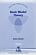Basic Model Theory (Kees Doets)

As the title indicates, this book introduces the reader to what is basic in model theory. A special feature is its use of the Ehrenfeucht game by which the reader is familiarised with the world of models.

•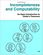Incompleteness and Computability: Gödel's Theorems

This book is an introduction to metamathematics and Gödel's Theorems. It covers recursive function theory, arithmetization of syntax, the first and second incompleteness theorem, models of arithmetic, second-order logic, and the lambda calculus.

Book Categories
 :All CategoriesTop Free BooksRecent BooksMiscellaneous BooksComputer EngineeringComputer LanguagesComputer ScienceData Science/DatabasesJava and Java EE (J2EE)Linux and UnixMathematicsMicrosoft and .NETMobile ComputingNetworking and CommunicationsSoftware EngineeringSpecial TopicsWeb Programming
Other Categories